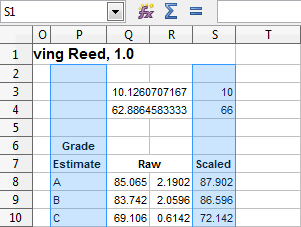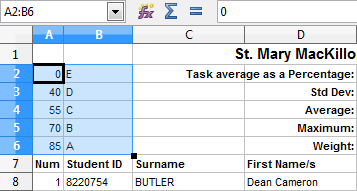13. Finishing the Content

In this lesson we’re going to finish off all the content for our “Summary” worksheet. There are two final columns needing attention, P and S:Just to give you some idea of what these columns are doing, Columns S is where we re-scale the raw marks to a new average and standard deviation. That’s what Cells “S3” and “S4” are used for, the values written there determine the column of scaled scores.

Column P is merely an estimate of the grade that each student would be getting according to their Raw score. That estimate is based on the following ranges:

0 - 39  = E
40 - 54  = D
55 - 69  = C
70 - 84  = B
85 - 100 = A

You could quite feasibly write a formula using IF statements to include those ranges, but then what if you wanted to change the range to produce more accurate grades? I will show you a method that involves looking up the grade from a table. Firstly though, let’s finish off Column S.

Column S
This column is basically the reverse of working out a Z-Score…this time we use the Z-Score we’ve already determined to generate a scaled score using new parameters. Remember that:

A “Z-Score” tells you how many standard deviations your result is away from the average.

With this in mind, we simply need to use all that information to generate a new score. Since Information Technology is primarily about problem solving, this is the perfect opportunity for you to do just that. If you really get stuck, ask questions!

Column P
This one requires the use of a new function called VLOOKUP. The VLOOKUP function basically looks up a value in a vertical list of sorted data (must be ascending), you can then tell it to return a matching piece of information in the row it found your search value. It’s probably easier to see it in action. Before we do though, we’re going to need a table of information to lookup the grade from, set up Cells “A2:B6” so they look like this:Now that we have a table to lookup the grade, let’s go back to Cell “P8“. In this cell we need to lookup the raw grade from the table pictured above, i.e:

VLOOKUP ( Q8 , A2:B6 , ............ )

You’ll notice that there isn’t a value that exactly matches the raw score of 85.065 but what VLOOKUP does is match the lookup to the highest value it did find, so in our case it would return the values in Row 6.

The missing portion of our formula above is where you request a specific column of data back from the VLOOKUP function. In our case we want Column 2 which is where the grade resides. The other thing needing attention is absolute cell references, when we refer to the lookup table, it needs to remain the same when we autofill this cell down, so:

VLOOKUP ( Q8 , A\$2:B\$6 , 2 )

What we’re basically saying with that formula is “Lookup Cell Q8 in the table A2:B6 and return the value you find in Column 2.

Conclusion
And there you have it! The structure and content of our Spreadsheet is complete. If you try deleting some rows of data from “gv” you should see the “Summary” sheet automatically readjust formulae to reflect the data it has…excellent!

We’re not quite done though…it’s time to make sure this sheet looks presentable and can be printed on one page!

2 thoughts on “13. Finishing the Content”

1.Jayden on said:

Where does the 10 and 66 from cells S3 and S4 come from? are you simply giving us these details or should these have been calculated some how?

•mrcruwys on said:

They are an example Average and Standard Deviation. Those two values are essentially available for the user of the spreadsheet to enter the expected Average and Standard Deviation for this sheet. So 66 and 10 are just an example…if you change it, your whole standardised column should reflect those alterations.